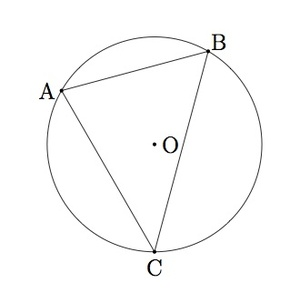# Inscribing a triangle in a circle

Alignments to Content Standards: G-C.A.3

The circumcenter of a triangle $ABC$ is the point in the plane which is equidistant from the three vertices $A$, $B$, and $C$.

1. If $O$ is the circumcenter of $\triangle ABC$, show that $\triangle ABC$ can be inscribed in a circle with center $O$.
2. Show that $\triangle ABC$ cannot have more than one circumcenter.

## IM Commentary

This problem introduces the circumcenter of a triangle and shows how it can be used to inscribe the triangle in a circle. It also shows that there cannot be more than one circumcenter. Note that while the construction of the circumcenter (using, e.g., compass and straightedge or dynamic geometry software) was not the focus of the task, the method for doing so is easily seen in the second half of the solution. This task could be used as a lead-in to an exercise performing such a construction.

In order to extend and enrich this task teachers may wish to have students experiment with many different triangles so that they see that there are three possibilities for the circumcenter $O$ of a given triangle:

• the point $O$ can be contained in the interior of the triangle
• the point $O$ can be contained on the boundary of the triangle
• the point $O$ can be on the exterior of the triangle

A natural place to go next with this problem is to classify the possibilities listed above in terms of the geometry of the triangle: that is the point $O$ is on the interior of the triangle when all three angles are acute, $O$ lies on the triangle when it is a right triangle, and $O$ is on the exterior of the triangle when the triangle has one obtuse angle.

This task can be used for assessment but it is primarily intended for instruction purposes as it aims at motiving why we wish to find the circumcenter of a triangle.

## Solution

1. Suppose $O$ is a point so that segments $\overline{OA}$, $\overline{OB}$, and $\overline{OC}$ are all congruent. Let $r = |OA|$ and consider the circle of radius $r$ with center $O$. The three vertices $A$, $B$, and $C$ all lie on the circle. Therefore the triangle $ABC$ consists in the union of three chords of the circle, $\overline{AB}$, $\overline{BC}$ and $\overline{AC}$, and so the triangle $ABC$ has been inscribed in a circle.

Below is a sample picture of a triangle $ABC$ with its circumcenter $O$ and the circle in which $\triangle ABC$ is inscribed:2. The point $O$ is equidistant from $A$ and $B$ and so this means that it lies on the perpendicular bisector $\ell_{AB}$ of $\overline{AB}$. The point $O$ is also equidistant from $B$ and $C$ and so lies on the perpendicular bisector $\ell_{BC}$ of $\overline{BC}$. The two lines $\ell_{AB}$ and $\ell_{BC}$ cannot be parallel (if they were parallel then the lines to which they are perpendicular, $\overleftrightarrow{AB}$ and $\overleftrightarrow{BC}$ would also be parallel) and so they meet in one point. This point of intersection of $\ell_{AB}$ and $\ell_{BC}$ is the only possible circumcenter for $\triangle ABC$. In order to show that this is the circumcenter of $\triangle ABC$ we would need to show that it also lies on the perpendicular bisector of $\overline {AC}$.

Alternatively, if $\triangle ABC$ had a second circumcenter, then it could be inscribed in a second, different circle. But two distinct circles can only meet in zero, one, or two points: these two distinct circumscribing circles would meet in three points which is not possible.